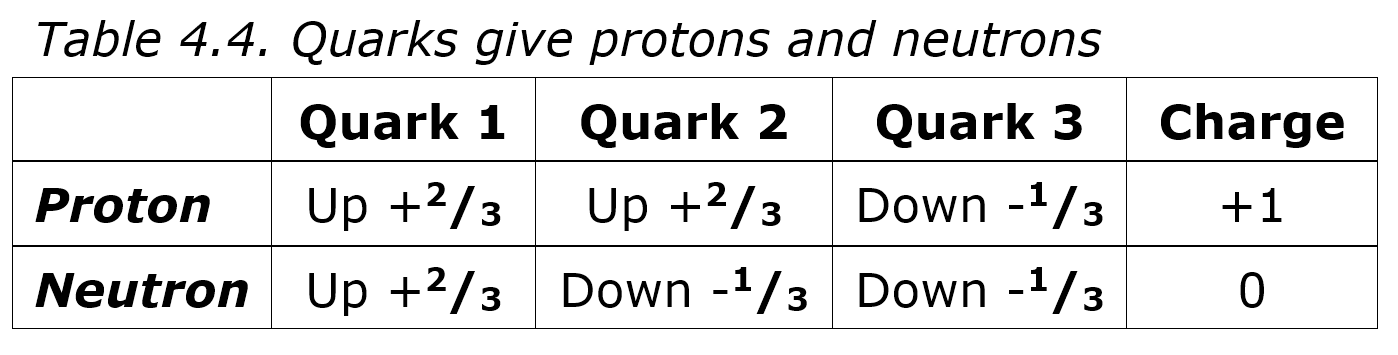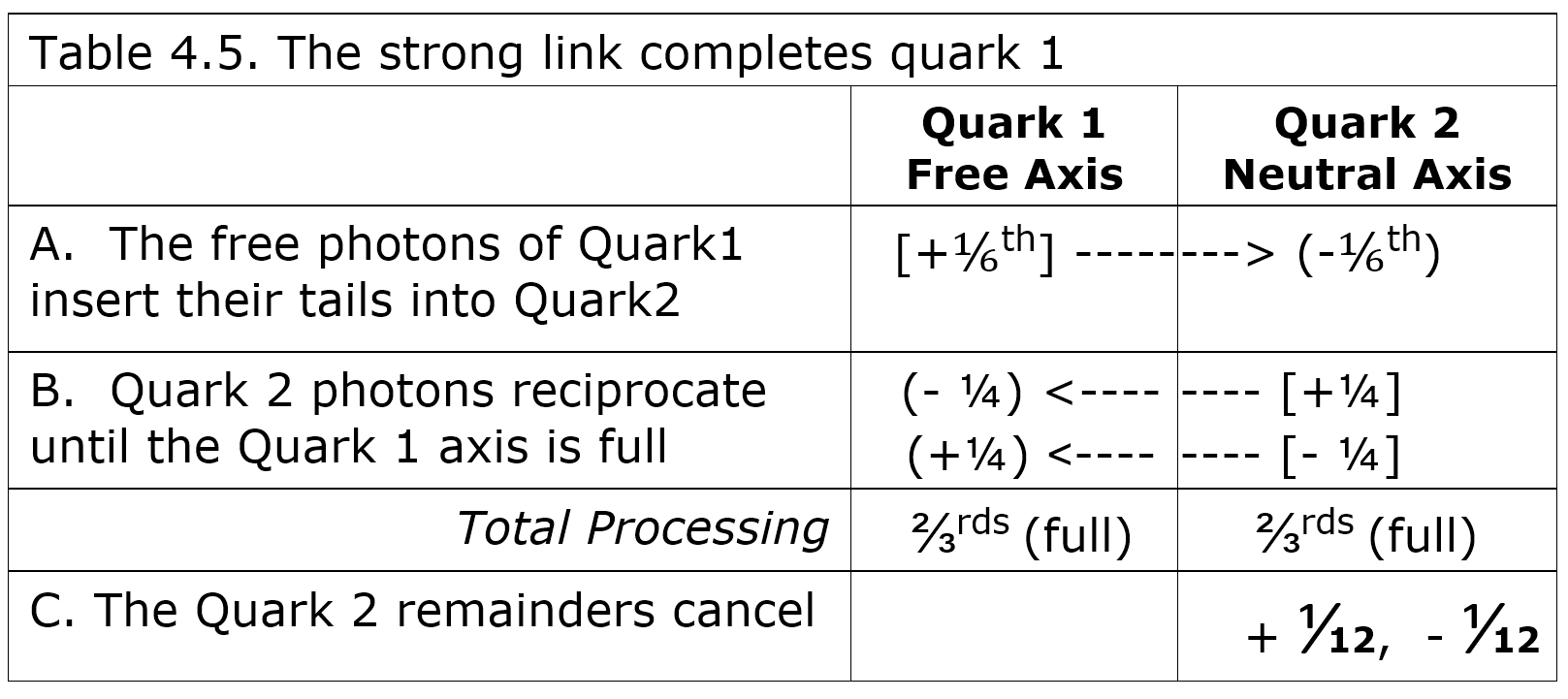# QR4.4.5 Protons and NeutronsThe atomic nucleus, once thought indivisible, is now known to consist of protons and neutrons that in turn are made up of quarks. A proton is two up quarks and a down quark and a neutron is two down quarks and an up, so the odd quark charges add nicely to give a positive proton and a neutral neutron (Table 4.4). In quantum processing terms, could quarks combine to give stable protons and neutrons?If the free photon “hooks” of one quark insert into the neutral axis of another quark, this gives a sixth of an axis of processing in both quarks (Table 4.5A), where one photon in two adjacent quarks uses all its processing with no remainder. The photons from the second quark’s neutral axis can return the favor until the first quark axis is full (Table 4.5B). Both axes are now complete and the positive and negative processing remaining in the neutral axis still cancels to neutral (Table 4.5C). Quark charge is unaffected because the charge axes aren’t involved so free-neutral photon sharing completes the free axis but the neutral axis is the same. Photon sharing binds quarks and creates the extra processing needed to stabilize the first quark by filling its free axis.